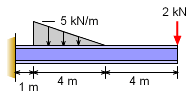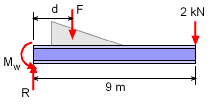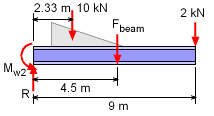Ch 4. Beam Stresses Multimedia Engineering Mechanics Bending Strainand Stress BeamDesign Shear Stress Built-upBeams
 Chapter 1. Stress/Strain 2. Torsion 3. Beam Shr/Moment 4. Beam Stresses 5. Beam Deflections 6. Beam-Advanced 7. Stress Analysis 8. Strain Analysis 9. Columns Appendix Basic Math Units Basic Equations Sections Material Properties Structural Shapes Beam Equations Search eBooks Dynamics Fluids Math Mechanics Statics Thermodynamics Author(s): Kurt Gramoll ©Kurt GramollMECHANICS - EXAMPLE ExampleCantilever Beam with Loads What is the lightest I-beam (S-shape section) that will support the loads shown without exceeding a bending stress of 150 MPa? Base beam selection on those in the appendix. of this eBook. Solution Design problems usually cannot be directly solved since the weight of the beam is not known at the start. Generally, the problem is first solved considering only the applied loads. Then, after the beam has been specified for the given loads, the weight of the beam will be added as another load and the problem is solved again. Sometimes this process takes 2 or 3 iterations but usually, it only takes 1.Free-Body Diagram (applied loads only) The beam needs to withstand the largest possible moment which will occur the wall since this is a cantilever beam. This moment will be      Mw = Mmax = Fd + 2(9)            = 0.5(5)(4) (4/3 + 1) + 18            = 23.33 + 18 = 41.33 kN-m The Section Modulus will be       Smin = Mw / σallow               = 41.33 / 150,000         = 275.5×10-6 m3         = 275.5×103 mm3 The lightest S-Shape I-beam using appendix Structural Shapes, is      S250×38Free-Body Diagram (with beam weight) However, now the problem needs to be solved again using the beam weight as an applied load. The new moment at the wall will be,      Mw-2 = 10(2.333) + 2(9) + 4.5(0.038)9            = 23.33 + 18 + 1.539 = 42.87 kN-m The added moment, 1.539 kN-m, is small and should not make a difference in the beam size selection. The new Section Modulus will be       Smin = Mw / σallow               = 42.87 / 150,000         = 285.8×103 mm3 The lightest beam is still S250×38

Practice Homework and Test problems now available in the 'Eng Mechanics' mobile app
Includes over 400 problems with complete detailed solutions.
Available now at the Google Play Store and Apple App Store.